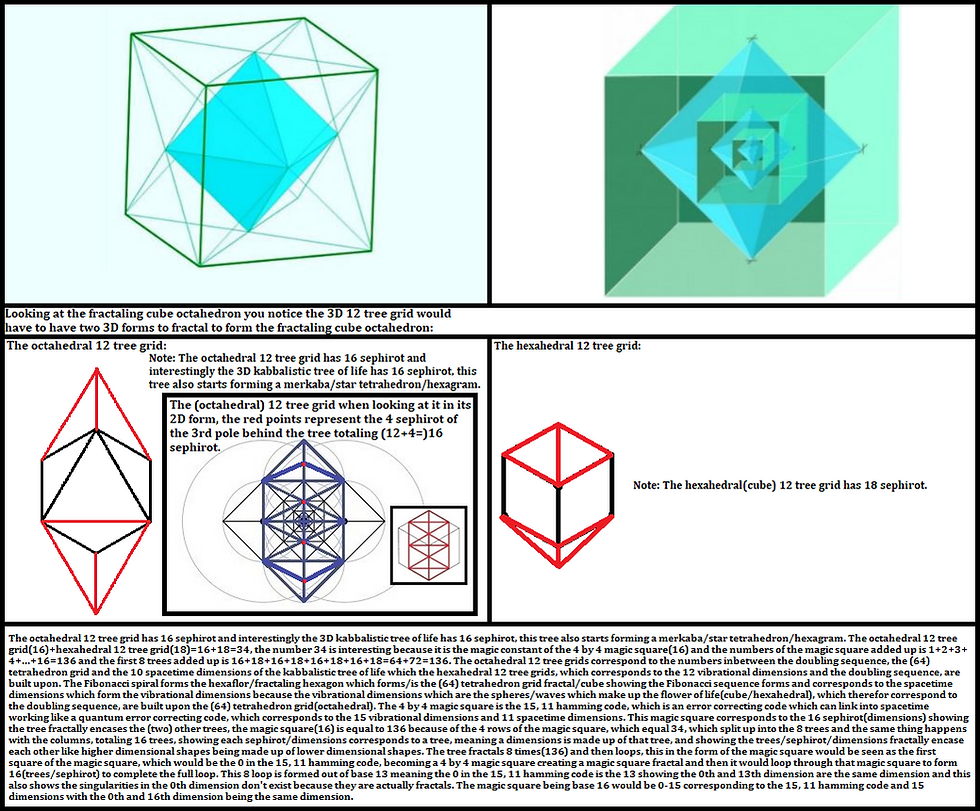top of page
Search

# The 3D 12 tree grid fractalThe octahedral 12 tree grid has 16 sephirot and interestingly the 3D kabbalistic tree of life has 16 sephirot, this tree also starts forming a merkaba/star tetrahedron/hexagram. The octahedral 12 tree grid(16)+hexahedral 12 tree grid(18)=16+18=34, the number 34 is interesting because it is the magic constant of the 4 by 4 magic square(16) and the numbers of the magic square added up is 1+2+3+4+...+16=136 and the first 8 trees added up is 16+18+16+18+16+18+16+18=64+72=136. The octahedral 12 tree grids correspond to the numbers inbetween the doubling sequence, the (64) tetrahedron grid and the 10 spacetime dimensions of the kabbalistic tree of life which the hexahedral 12 tree grids, which corresponds to the 12 vibrational dimensions and the doubling sequence, are built upon. The Fibonacci spiral forms the hexaflor/fractaling hexagon which forms/is the (64) tetrahedron grid fractal/cube showing the Fibonacci sequence forms and corresponds to the spacetime dimensions which form the vibrational dimensions because the vibrational dimensions which are the spheres/waves which make up the flower of life(cube/hexahedral), which therefor correspond to the doubling sequence, are built upon the (64) tetrahedron grid(octahedral). The 4 by 4 magic square is the 15, 11 hamming code, which is an error correcting code which can link into spacetime working like a quantum error correcting code, which corresponds to the 15 vibrational dimensions and 11 spacetime dimensions. This magic square corresponds to the 16 sephirot(dimensions) showing the tree fractally encases the (two) other trees, the magic square(16) is equal to 136 because of the 4 rows of the magic square, which equal 34, which split up into the 8 trees and the same thing happens with the columns, totaling 16 trees, showing each sephirot/dimensions corresponds to a tree, meaning a dimensions is made up of that tree, and showing the trees/sephirot/dimensions fractally encase each other like higher dimensional shapes being made up of lower dimensional shapes. The tree fractals 8 times(136) and then loops, this in the form of the magic square would be seen as the first square of the magic square, which would be the 0 in the 15, 11 hamming code, becoming a 4 by 4 magic square creating a magic square fractal and then it would loop through that magic square to form 16(trees/sephirot) to complete the full loop. This 8 loop is formed out of base 13 meaning the 0 in the 15, 11 hamming code is the 13 showing the 0th and 13th dimension are the same dimension and this also shows the singularities in the 0th dimension don't exist because they are actually fractals. The magic square being base 16 would be 0-15 corresponding to the 15, 11 hamming code and 15 dimensions with the 0th and 16th dimension being the same dimension.